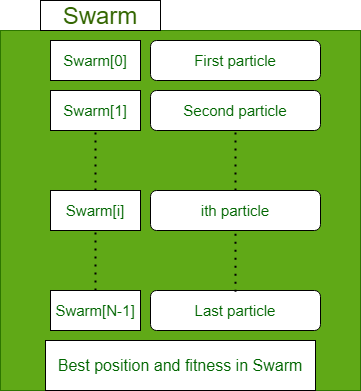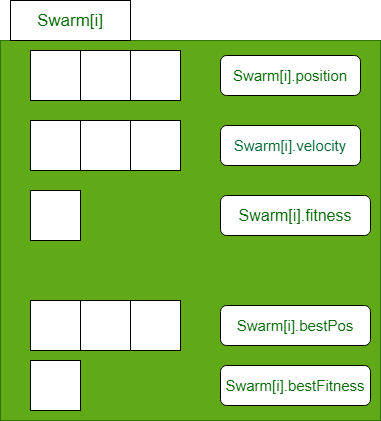Skip to content
Related Articles
Particle Swarm Optimization (PSO) – An Overview
• Last Updated : 03 May, 2021

The process of finding optimal values for the specific parameters of a given system to fulfill all design requirements while considering the lowest possible cost is referred to as an optimization. Optimization problems can be found in all fields of science.

Conventional optimization algorithms (Deterministic algorithms) have some limitations such as:

• single-based solutions
• converging to local optima
• unknown search space issues

To overcome these limitations, many scholars and researchers have developed several metaheuristics to address complex/unsolved optimization problems. Example: Particle Swarm Optimization, Grey wolf optimization, Ant colony Optimization, Genetic Algorithms, Cuckoo search algorithm etc.

The Introduction to Particle Swarm Optimization (PSO) article explained the basics of stochastic optimization algorithms and explained the intuition behind particle swarm optimization (PSO). This article aims to deep dive into particle swarm optimization (PSO).

## Inspiration of the algorithm

Particle Swarm Optimization (PSO) is a powerful meta-heuristic optimization algorithm and inspired by swarm behavior observed in nature such as fish and bird schooling.  PSO is a Simulation of a simplified social system. The original intent of PSO algorithm was to graphically simulate the graceful but unpredictable choreography of a bird flock.

In nature, any of the bird’s observable vicinity is limited to some range. However, having more than one birds allows all the birds in a swarm to be aware of the larger surface of a fitness function.

Let’s mathematically model, the above-mentioned principles to make the swarm find the global minima of a fitness function

## Mathematical model

• Each particle in particle swarm optimization has an associated position, velocity, fitness value.
• Each particle keeps track of the particle_bestFitness_value particle_bestFitness_position.
• A record of global_bestFitness_position and global_bestFitness_value is maintained.Fig 1: Data structure to store Swarm populationFig 2: Data structure to store ith particle of Swarm

## Algorithm

Parameters of problem:

• Number of dimensions (d)
• Lower bound (minx)
• Upper bound (maxx)

Hyperparameters of the algorithm:

• Number of particles (N)
• Maximum number of iterations (max_iter)
• Inertia (w)
• Cognition of particle (C1)
• Social influence of swarm (C2)

Algorithm:

```Step1: Randomly initialize Swarm population of N particles Xi ( i=1, 2, …, n)
Step2: Select hyperparameter values
w, c1 and c2
Step 3: For Iter in range(max_iter):  # loop max_iter times
For i in range(N):  # for each particle:
a. Compute new velocity of ith particle
swarm[i].velocity =
w*swarm[i].velocity +
r1*c1*(swarm[i].bestPos - swarm[i].position) +
r2*c2*( best_pos_swarm - swarm[i].positin)
b. If velocity is not in range [minx, max] then clip it
if swarm[i].velocity < minx:
swarm[i].velocity = minx
elif swarm[i].velocity[k] > maxx:
swarm[i].velocity[k] = maxx
c. Compute new position of ith particle using its new velocity
swarm[i].position += swarm[i].velocity
d. Update new best of this particle and new best of Swarm
if swarm[i].fitness < swarm[i].bestFitness:
swarm[i].bestFitness = swarm[i].fitness
swarm[i].bestPos = swarm[i].position

if swarm[i].fitness < best_fitness_swarm
best_fitness_swarm = swarm[i].fitness
best_pos_swarm = swarm[i].position
End-for
End -for
Step 4: Return best particle of Swarm```My Personal Notes arrow_drop_up